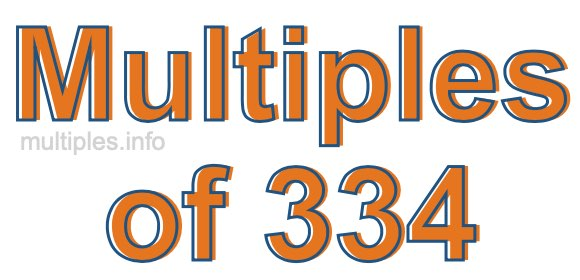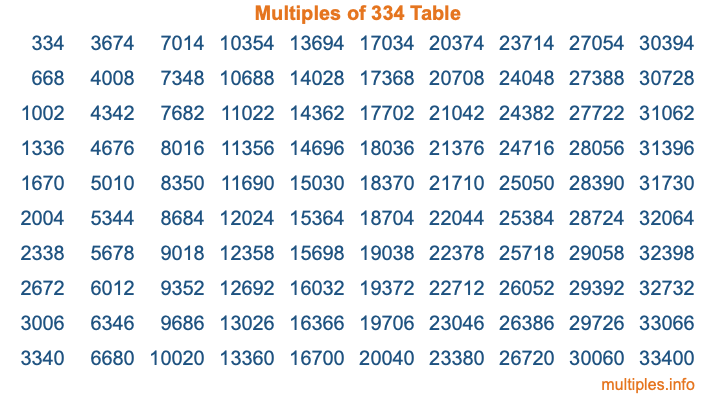Multiples of 334Welcome to the Multiples of 334 page. Here we will first teach you everything you will ever need to know about the multiples of 334, and then give you a study guide summary of everything we taught you to make sure you remember it all. Use this page to look up facts and learn information about the multiples of 334. This page will make you a multiples of three hundred thirty-four expert!

Definition of Multiples of 334
Multiples of 334 are all the numbers that when divided by 334 equal an integer. Each of the multiples of 334 are called a multiple. A multiple of 334 is created by multiplying 334 by an integer.

Therefore, to create a list of multiples of 334, you start with 1 multiplied by 334, then 2 multiplied by 334, then 3 multiplied by 334, and so on for as long as you want. Thus, the list of the first five multiples of 334 is 334, 668, 1002, 1336, and 1670. To see a larger list of multiples of 334, see the printable image of Multiples of 334 further down on this page. We also have a category where you can choose any nth multiple of 334.

Multiples of 334 Checker
The Multiples of 334 Checker below checks to see if any number of your choice is a multiple of 334. In other words, it checks to see if there is any number (integer) that when multiplied by 334 will equal your number. To do that, we divide your number by 334. If the the quotient is an integer, then your number is a multiple of 334.

Is  a multiple of 334?

Least Common Multiple of 334 and ...
A Least Common Multiple (LCM) is the lowest multiple that two or more numbers have in common. This is also called the smallest common multiple or lowest common multiple and is useful to know when you are adding our subtracting fractions. Enter one or more numbers below (334 is already entered) to find the LCM.

Check out our LCM Calculator if you need more details about the Least Common Multiple or if you need the LCM for different numbers for adding and subtraction fractions.

nth Multiple of 334
As we stated above, 334 is the first multiple of 334, 668 is the second multiple of 334, 1002 is the third multiple of 334, and so on. Enter a number below to find the nth multiple of 334.

th multiple of 334

Multiples of 334 vs Factors of 334
334 is a multiple of 334 and a factor of 334, but that is where the similarities end. All postive multiples of 334 are 334 or greater than 334. All positive factors of 334 are 334 or less than 334.

Below is the beginning list of multiples of 334 and the factors of 334 so you can compare:

Multiples of 334: 334, 668, 1002, 1336, 1670, etc.

Factors of 334: 1, 2, 167, 334

As you can see, the multiples of 334 are all the numbers that you can divide by 334 to get a whole number. The factors of 334, on the other hand, are all the whole numbers that you can multiply by another whole number to get 334.

It's also interesting to note that if a number (x) is a factor of 334, then 334 will also be a multiple of that number (x).

Multiples of 334 vs Divisors of 334
The divisors of 334 are all the integers that 334 can be divided by evenly. Below is a list of the divisors of 334.

Divisors of 334: 1, 2, 167, 334

The interesting thing to note here is that if you take any multiple of 334 and divide it by a divisor of 334, you will see that the quotient is an integer.

Multiples of 334 Table
Below is an image of the first 100 multiples of 334 in a table. The table is in chronological order, column by column. The first column has the first ten multiples of 334, the second column has the next ten multiples of 334, and so on.The Multiples of 334 Table is also referred to as the 334 Times Table or Times Table of 334. You are welcome to print out our table for your studies.

Negative Multiples of 334
Although not often discussed or needed in math, it is worth mentioning that you can make a list of negative multiples of 334 by multiplying 334 by -1, then by -2, then by -3, and so on, to get the following list of negative multiples of 334:

-334, -668, -1002, -1336, -1670, etc.

Multiples of 334 Summary
Below is a summary of important Multiples of 334 facts that we have discussed on this page. To retain the knowledge on this page, we recommend that you read through the summary and explain to yourself or a study partner why they hold true.

There are an infinite number of multiples of 334.

A multiple of 334 divided by 334 will equal a whole number.

334 divided by a factor of 334 equals a divisor of 334.

The nth multiple of 334 is n times 334.

The largest factor of 334 is equal to the first positive multiple of 334.

334 is a multiple of every factor of 334.

334 is a multiple of 334.

A multiple of 334 divided by a divisor of 334 equals an integer.

334 divided by a divisor of 334 equals a factor of 334.

Any integer times 334 will equal a multiple of 334.

Multiples of a Number
Here you can get the multiples of another number, all with the same attention to detail as we did for multiples of 334 on this page.

Multiples of
Multiples of 335
Did you find our page about multiples of three hundred thirty-four educational? Do you want more knowledge? Check out the multiples of the next number on our list!